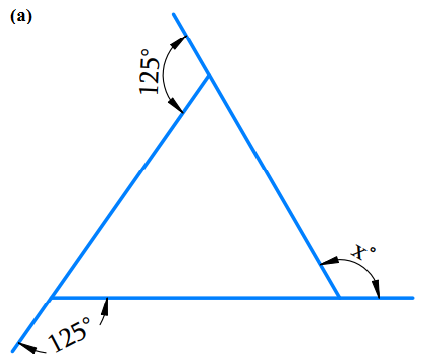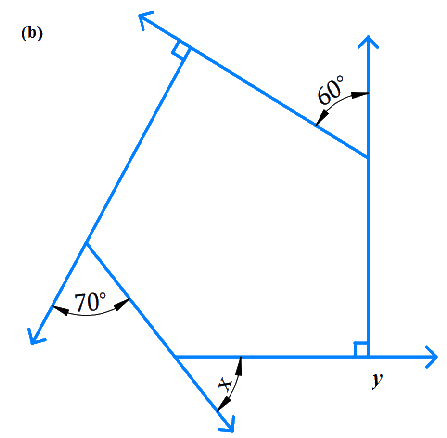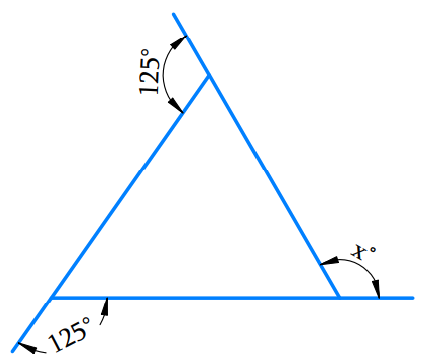# Excercise 3.2 Understanding Quadrilaterals- NCERT Solutions Class 8

## Chapter 3 Ex.3.2 Question 1

Find $$x$$ in the following figures.### Solution

What is Known?

Other angles of given polygon.

What is Unknown?

Angle $$x$$ in the above figures $$a$$ and $$b$$.

Reasoning:

We know that the sum of the measures of the exterior angles of any polygon is $$360^\circ$$ So equate all the angle sum to $$360^\circ$$ and find out the unknown angle.

Steps:

(a)Sum of the measures of the external angles,

\begin{align}125^\circ \, + 125^\circ \, + \,x\, = \,360^\circ \\{\rm{25}}0^\circ + {\rm{ }}x{\rm{ }} = {\rm{ 36}}0^\circ \\x{\rm{ }} = {\rm{ 11}}0^\circ\end{align}

(b)\begin{align}y &= {{{180}^{\rm{\circ}}} - {{90}^{\rm{\circ}}}\quad {\text{[linear pair angles]}}}\\y &= {{90}^{\rm{\circ}}}\end{align}

Sum of the measures of the external angles is $$360^\circ$$,

\begin{align}60^\circ + 90^\circ + 70^\circ + {\rm{ }}x{\rm{ }} + y &= {360^{\rm{\circ}}}\\ {\rm{6}}0^\circ + {\rm{9}}0^\circ + {\rm{7}}0^\circ + {\rm{ }}x{\rm{ }} + {\rm{ 9}}0^\circ &= {\rm{36}}0^\circ \\{\rm{31}}0^\circ + x &= {\rm{36}}0^\circ \\x &= {\rm{5}}0^\circ\end{align}

## Chapter 3 Ex.3.2 Question 2

Find the measure of each exterior angle of a regular polygon of

(i) $$9$$ sides

(ii) $$15$$ sides

### Solution

What is Known?

The number of sides of the polygon.

What is Unknown?

Exterior angle of a regular polygon of $$9$$ sides.

Exterior angle of a regular polygon of $$15$$ sides.

Reasoning:

Irrespective of the number of sides of the polygon, the measure of the exterior angles is equal and the sum of the measure of all the exterior angles of the regular polygon is equal to $$360^\circ$$.

Steps:

(i) $$9$$ sides

Total measure of all exterior angles $$= {360^{\rm{\circ}}}$$

Each exterior angle

\begin{align}&= \frac{{{\text{sum of exterior angle}}}}{{{\text{number of sides}}}}\\&= \,\,\frac{{{{360}^{\rm{\circ}}}}}{9}\\&= \,\,{40^{\rm{\circ}}}\end{align}

Each exterior angle $$={\rm{4}}0^\circ$$

(ii) $$15$$ sides

Total measure of all exterior angles $$= 360^\circ$$

Each exterior angle

\begin{align}&= \frac{{{\text{sum of exterior angle}}}}{{{\text{number of sides}}}}\\&= \frac{{{{360}^{\rm{\circ}}}}}{{15}}\\&= \,{24^{\rm{\circ}}}\end{align}

Each exterior angle $$= 24^\circ$$

## Chapter 3 Ex.3.2 Question 3

How many sides does a regular polygon have if the measure of an exterior angle is $$24^\circ$$?

### Solution

What is Known?

Measure of an exterior angle is $$24^\circ$$.

What is Unknown?

The number of sides of the regular polygon.

Steps:

Total measure of all the exterior angles of the regular polygon $$= 360^\circ$$

Let number of sides be $$= n$$.

Measure of each exterior angle $$= 24^\circ$$

Therefore, the number of sides

\begin{align} &= \,\,\frac{{{\text{Sum of exterior angles}}}}{{{\text{Each exterior angle}}}}\\&= \,\,\,\frac{{360^\circ }}{{24^\circ }}\\&= \,\,\,15\end{align}

$$\therefore$$ $$R$$, regular polygon has $$15$$ sides.

## Chapter 3 Ex.3.2 Question 4

How many sides does a regular polygon have if each of its interior angles is $$165^\circ$$?

### Solution

What is Known?

Measure of an interior angle is $$165^\circ$$.

What is Unknown?

The number of sides of the regular polygon.

Reasoning:

We know that:

a) Irrespective of the number of sides of the polygon, the measure of the exterior angles is equal and the sum of the measures of all the exterior angles of the regular polygon is equal to $$360°$$.

b) The measure of interior angle of regular polygon is $$\rm{}\,(n-2) *180/n$$, where ‘$$n$$’ is the number of sides of the polygon

Steps:

Let number of sides be $$\rm n$$.

Measure of each interior angle $$= {\rm{16}}{{\rm{5}}^{\rm{\circ}}}$$

Measure of each exterior angle $$={\rm{18}}0^\circ {\rm{ }} - {\rm{ 165}}^\circ {\rm{ }} = {\rm{ 15}}^\circ$$ [linear pair angles]

Number of sides

\begin{align}&= \,\,\frac{{{\text{Sum of exterior angles}}}}{{{\text{Each exterior angle}}}}\\&={} \,\,\frac{{{\rm{36}}0^\circ }}{{{\rm{15}}^\circ }}\\&= \,\,\,24\end{align}

Hence, the regular polygon has $$24$$ sides.

## Chapter 3 Ex.3.2 Question 5

(a) Is it possible to have a regular polygon with measure of each exterior angle as $$22^\circ$$?

(b)Can it be an interior angle of a regular polygon? Why?

### Solution

What is Known?

Measure of an exterior angle is $$22^\circ$$.

What is Unknown?

To find whether a regular polygon with exterior angle $$= 22^\circ$$ is possible or not.

Reasoning:

Number of sides of any polygon

\begin{align}\,{\text{ }}&= \,\frac{{{\rm{36}}{{\rm{0}}^{\rm{\circ}}}}}{{{\text{Exterior angle}}}}\end{align}

where the answer is whole number .

Steps:

(a) Is it possible to have a regular polygon with measure of each exterior angle as $$22^\circ$$?

Total measure of all exterior angles $$= {\rm{36}}0^\circ$$

Let number of sides be $$= \rm n$$. Measure of each exterior angle $$= {\rm{22}}^\circ$$

Therefore, the number of sides

\begin{align}&=\,\frac{{{\text{Sum of exterior angles}}}}{{{\text{Each exterior angle}}}}\\&= \frac{{{{360}^{\rm{o}}}}}{{{{22}^{\rm{o}}}}}\\&= 16.36\end{align}

We cannot have regular polygon with each exterior angle = $$22^\circ$$ as the number of sides is not a  whole number [ $$22$$ is not a perfect divisor of $$360^\circ$$].

(b) Can it be an interior angle of a regular polygon? Why?

Measure of each interior angle $$= 22^\circ$$

Measure of each exterior angle

\begin{align}\\&= 180^\circ - {\rm{22}}^\circ {\rm{ }}\\&= {\rm{158}}^\circ\end{align}

Number of sides

\begin{align}&= \frac{{{\text{Sum of exterior angles}}}}{{{\text{Each exterior angle}}}}\\&= \frac{{{\rm{36}}0^\circ }}{{{\rm{158}}^\circ }}\\&= 2.27\end{align}

We cannot have regular polygon with each interior angle as $$22^\circ$$ because the number of sides is not a whole number[ $$22$$ is not a perfect divisor of $$360^\circ$$].

## Chapter 3 Ex.3.2 Question 6

(a) What is the minimum interior angle possible for a regular polygon? Why?

(b) What is the maximum exterior angle possible for a regular polygon?

### Solution

What is Known?

We know polygons according to the number of sides (or vertices) they have.

What is Unknown?

Minimum interior angle possible for a regular polygon.

Maximum exterior angle possible for a regular polygon.

Reasoning:We know that the sum of measure of interior angle of triangle is  $$={\rm{18}}0^\circ$$

Equilateral triangle is regular polygon having maximum exterior angle because it consists of least number of sides.

Steps:

(a) What is the minimum interior angle possible for a regular polygon? Why?

Consider a regular polygon having the least number of sides (i.e., an equilateral triangle).

We know Sum of all the angles of a triangle $$= {\rm{18}}0^\circ$$

\begin{align}x + x + x{\rm{ }}& = {\rm{ 18}}0^\circ \\{\rm{3}}x &= {\rm{18}}0^\circ \\x &= \frac{{{\rm{18}}0^\circ }}{3}\\x &= {\rm{6}}0^\circ\end{align}Thus, minimum interior angle possible for a regular polygon$$= 60^\circ$$

(b) What is the maximum exterior angle possible for a regular polygon?

We know that the exterior angle and an interior angle will always form a linear pair. Thus exterior angle will be maximum when interior angle is minimum.

Exterior angle

\begin{align}&= {\rm{18}}{0^{\rm{\circ}}} - \,\,{\rm{6}}0^\circ \\&={120^{\rm{\circ}}}.\end{align}

Therefore, maximum exterior angle possible for a regular polygon is $$120^\circ$$

Equilateral triangle is a regular polygon having maximum exterior angle because it consists of least number of sides.

Related Sections
Related Sections
Instant doubt clearing with Cuemath Advanced Math Program